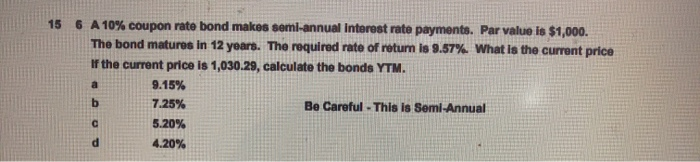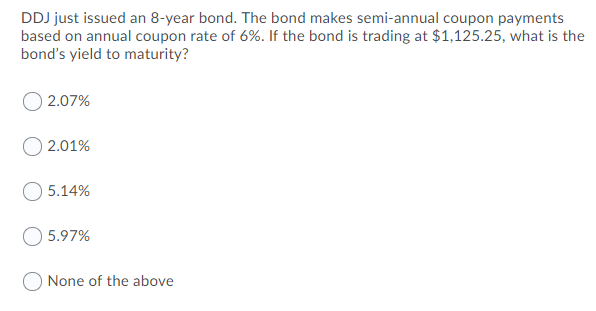Question

# A 10-year corporate bond has a coupon rate of 6% with semi-annual payments. If the current...

A 10-year corporate bond has a coupon rate of 6% with semi-annual payments. If the current value of the bond in the marketplace is \$900, then what is the Yield-to-Maturity (YTM)? How to do this on financial calculator?

Yield-to-Maturity (YTM) of the Bond

The Yield to maturity of (YTM) of the Bond is calculated using financial calculator as follows (Normally, the YTM is calculated either using EXCEL Functions or by using Financial Calculator)

 Variables Financial Calculator Keys Figure Par Value/Face Value of the Bond [\$1,000] FV 1,000 Coupon Amount [\$1,000 x 6.00% x ½] PMT 30 Market Interest Rate or Yield to maturity on the Bond 1/Y ? Maturity Period/Time to Maturity [10 Years x 2] N 20 Bond Price [-\$900] PV -900

We need to set the above figures into the financial calculator to find out the Yield to Maturity of the Bond. After entering the above keys in the financial calculator, we get the semi-annual yield to maturity (YTM) on the bond = 3.72%.

The semi-annual Yield to maturity = 3.72%.

Therefore, the annual Yield to Maturity of the Bond = 7.44% [3.72% x 2]

“Hence, the Yield-to-Maturity (YTM) of the Bond will be 7.44%”

#### Earn Coins

Coins can be redeemed for fabulous gifts.

Similar Homework Help Questions
• ### ABC issued 12-year bonds at a coupon rate of 8% with semi-annual payments. If the bond...

ABC issued 12-year bonds at a coupon rate of 8% with semi-annual payments. If the bond currently sells for \$1050 of par value, what is the YTM? ABC issued 12-year bonds 2 years ago at a coupon rate of 8% with semi-annual payments. If the bond currently sells for 105% of par value, what is the YTM? A bond has a quoted price of \$1,080.42. It has a face value of \$1000, a semi-annual coupon of \$30, and a maturity...

• ### Five years ago, Cookie Corp. issued a bond with 15% coupon rate, semi-annual coupon payments, \$1000...

Five years ago, Cookie Corp. issued a bond with 15% coupon rate, semi-annual coupon payments, \$1000 face value and 15 years until maturity. The current YTM is 16%. If you sell the bond today (next coupon payment is in 6 months from today), after having owned it for 4 years, what would your capital gain/loss yield? Please show formulas and do not use excel or financial calculator.

• ### What is the current value of a 10-year semi-annual interest bond that has a \$1,000 maturity...

What is the current value of a 10-year semi-annual interest bond that has a \$1,000 maturity value, coupon rate of 10%, and the YTM is 10%? a.)       \$376.09 b.)      \$623.11 c.)       \$1,000.00 d.)      \$692.77 What is the current value of a 10-year semi-annual interest bond that has a \$1,000 maturity value, coupon rate of 6%, and the YTM is 10%? a.)       \$750.76 b.)      \$376.89 c.)       \$373.87 d.)      \$1,000.00

• ### Five years ago, Cookie Limited issued a bond with 15% coupon rate, semi-annual coupon payments, \$1000...

Five years ago, Cookie Limited issued a bond with 15% coupon rate, semi-annual coupon payments, \$1000 face value and 15 years until maturity. If you bought this bond 4 years ago (right after the bond made its coupon payment) when the YTM was 11%, how much did you pay for the bond? Please do not use excel or a financial calculator and show formulas.

• ### A semi-annual coupon bond has a 6 percent coupon rate, a \$1,000 face value, a current...

A semi-annual coupon bond has a 6 percent coupon rate, a \$1,000 face value, a current value of \$1,036.09, and 3 years until the first call date. What is the call price if the yield to call is 6.5 percent? A STRIPS has a yield to maturity of 6.2 percent, a par value of \$25,000, and a time to maturity of 10 years. What is the price

• ### 15 6 A 10% coupon rate bond makes semi-annual Interest rate payments. Par value is \$1,000....15 6 A 10% coupon rate bond makes semi-annual Interest rate payments. Par value is \$1,000. The bond matures in 12 years. The required rate of return is 9.57%. What is the current price If the current price is 1,030.29, calculate the bonds YTM. a 9.15% b 7.25% Be Careful - This is Semi-Annual 5.20% 4.20% с

• ### A corporation has an outstanding bond with the following characteristics: Coupon Rate 6.0% Interest Payments Semi-Annually...

A corporation has an outstanding bond with the following characteristics: Coupon Rate 6.0% Interest Payments Semi-Annually Face Value \$1,000 Years to maturity 20 Current market value \$854.64 What is the yield to maturity (YTM) for this bond? **Please show how you got the answer**

• ### Bond A is a semi-annual coupon bond that has a face value of \$1000, a 10% coupon rate

Bond A is a semi-annual coupon bond that has a face value of \$1000, a 10% coupon rate, a five year maturity, and a yield to maturity of 7%. At the maturity date, how much payment should the bond investor expect from the bond? (a) \$50 (b) \$100 (c) \$1035 (d) \$1050

• ### A 5.5%, 5-year bond with semi-annual coupon payments and a face value of \$1,000 has a...

A 5.5%, 5-year bond with semi-annual coupon payments and a face value of \$1,000 has a market price of \$1,032.19. Assume that the next coupon payment is exactly six months away. a) What is the yield-to-maturity of the bond? b) What is the effective annual rate implied by this price?

• ### DDJ just issued an 8-year bond. The bond makes semi-annual coupon payments based on annual coupon...DDJ just issued an 8-year bond. The bond makes semi-annual coupon payments based on annual coupon rate of 6%. If the bond is trading at \$1,125.25, what is the bond's yield to maturity? 2.07% 2.01% 5.14% 5.97% None of the above